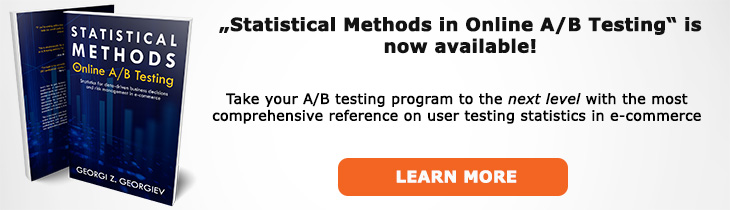# What does "Composite Hypothesis" mean?

Definition of Composite Hypothesis in the context of A/B testing (online controlled experiments).

## What is a Composite Hypothesis?

In hypothesis testing a composite hypothesis is a hypothesis which covers a set of values from the parameter space. For example, if the entire parameter space covers -∞ to +∞ a composite hypothesis could be μ ≤ 0. It could be any other number as well, such 1, 2 or 3,1245. The alternative hypothesis is always a composite hypothesis: either one-sided hypothesis if the null is composite or a two-sided one if the null is a point null. The "composite" part means that such a hypothesis is the union of many simple point hypotheses.

In a Null Hypothesis Statistical Test only the null hypothesis can be a point hypothesis. Also, a composite hypothesis usually spans from -∞ to zero or some value of practical significance or from such a value to +∞.

Testing a composite null is what is most often of interest in an A/B testing scenario as we are usually interested in detecting and estimating effects in only one direction: either an increase in conversion rate or average revenue per user, or a decrease in unsubscribe events would be of interest and not its opposite. In fact, running a test so long as to detect a statistically significant negative outcome can result in significant business harm.

## Articles on Composite Hypothesis

Like this glossary entry? For an in-depth and comprehensive reading on A/B testing stats, check out the book "Statistical Methods in Online A/B Testing" by the author of this glossary, Georgi Georgiev.## Glossary Index by Letter

Select a letter to see all A/B testing terms starting with that letter or visit the Glossary homepage to see all.